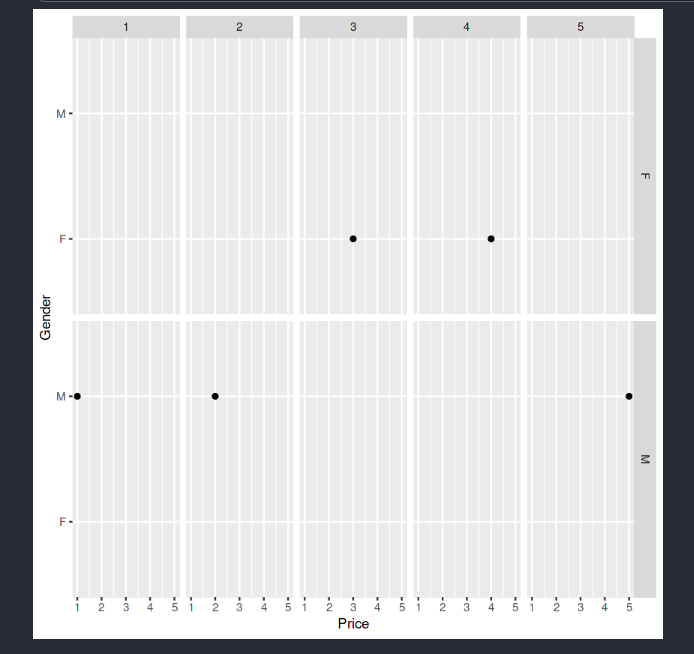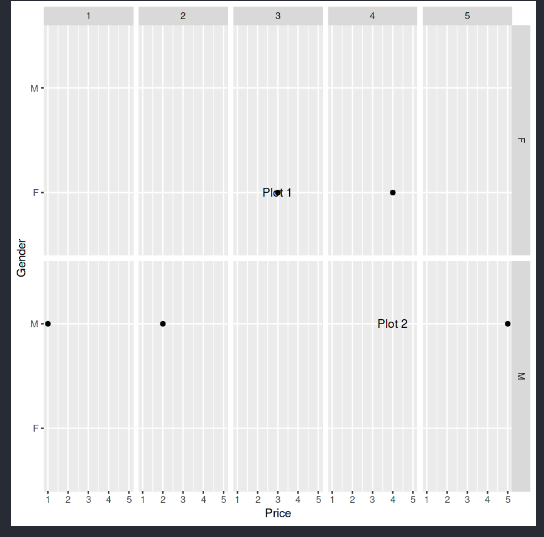# Annotating text on individual facet in ggplot2 in R

• Last Updated : 23 Aug, 2021

In this article, we will discuss how to annotate a text on the Individual facet in ggplot2 in R Programming Language.

To plot facet in R programming language, we use the facet_grid() function from the ggplot2 library. The facet_grid() is used to form a matrix of panels defined by row and column faceting variables, i.e. to display all combinations of the variables that exist in the data. Annotating text is somewhat similar to label text, but there is a difference between a label and annotating is that we generally can’t change the position and properties of a label but in annotating a text there is no such predefined rule. Annotating is additional metadata about the figure, and a label is a piece of information about the figure used to identify the figure.

Syntax: facet_grid(rows = NULL,cols = NULL,scales = “fixed”,space = “fixed”,shrink = TRUE,..)

Parameters:

• rows, cols: Used for defining faceting groups on the rows or columns dimension.
• scales: To set scales sharing across all facets. The default is “fixed” which means all scales are shared across all facets. If they vary across rows then scales are set to be “free_x”, to vary across columns it is set to be “free_y”, and for both rows and columns, it is set to be “free”.
• space: To set the size of the panel. By default, it is set to be “fixed” i.e. all panels have the same size. If it is set to be “free_y” then their height will be proportional to the length of the y scale, if it is set to be  “free_x” then their width will be proportional to the length of the x scale and if it is set to be “free” then both height and width will vary.
• shrink: It is used to fit the output of the statistics result to the screen, by default it is true.

First, load and install the necessary libraries need to plot the facet, i.e. load the ggplot2 library and create a dummy dataset.

Dataset in use:

```   Name Gender Price
1     A      M     1
2     B      M     2
3     C      F     3
4     D      F     4
5     E      M     5```

Plot the data using ggplot command by passing the data frame as an argument. Let us first look at the plot without being annotated, so that the difference is apparent.

Example: Plot without annotation text on individual facets.

## R

 `# Facet before adding Annotating text `` ` `# installing ggplot2 library``install.packages``(``"ggplot2"``)`` ` `# loading the library``library``(``"ggplot2"``)`` ` `# creating a dummy dataset``people <- ``c``(``"A"``,``"B"``,``"C"``,``"D"``,``"E"``)``gender <- ``c``(``"M"``,``"M"``,``"F"``,``"F"``,``"M"``)    ``price <- ``c``(1,2,3,4,5)`` ` `df <- ``data.frame``(Name = people, Gender = gender, Price = price)`` ` `# plotting the data using ggplot``plt <- ``ggplot``(``     ` `    ``# providing dataset``    ``df,``     ` `    ``# to plot price vs Gender ``    ``aes``(x=Price, y=Gender)``     ` `    ``# to plot data point as a (.) dot``) + ``geom_point``()`` ` `# creating facet from Gender and Price variable``plt <- plt + ``facet_grid``(Gender ~ Price)`` ` `# displaying facet plot``plt`

Output:To add text, create custom annotated text that you want to apply to the plot.

Example:

ann_dat_text<-data.frame(

Gender=c(“F”,”M”),

Price=c(3,4),

label=c(“Plot 1″,”Plot 2”)

)

Here, in the above statement, we are creating a data frame that we are going to use for annotating the text onto the facet. Here we are applying the label “Plot 1” and “Plot 2” onto both facets, on the “F” side of the Gender Column we will be having annotated text as “Plot 1” and on the “M” side we will be having an annotated text “Plot 2”. And Price will specify the position of the text.

Now apply this custom text to the facets by passing them within geom_text() function with appropriate arguments supplied with required values.

Syntax:

geom_text( data, label)

Using facet_grid, we have created two facets and in geom_text() the data will be the newly created custom annotated data that we are going to use to apply annotated text onto the facet.

Example: Plot after annotating individual facets.

## R

 `# Annotating text on individual facet in ggplot2`` ` `# installing ggplot2 library``install.packages``(``"ggplot2"``)`` ` `# loading the library``library``(``"ggplot2"``)`` ` `# creating a dummy dataset``people <- ``c``(``"A"``,``"B"``,``"C"``,``"D"``,``"E"``)``gender <- ``c``(``"M"``,``"M"``,``"F"``,``"F"``,``"M"``)    ``price <- ``c``(1,2,3,4,5)`` ` `df <- ``data.frame``(Name = people, Gender = gender, Price = price)`` ` `# plotting the data using ggplot``plt <- ``ggplot``(``     ` `    ``# providing dataset``    ``df,``     ` `    ``# to plot price vs Gender ``    ``aes``(x=Price, y=Gender)``     ` `    ``# to plot data point as a (.) dot``) + ``geom_point``()`` ` ` ` `# creating a dataframe for annotating text``ann_dat_text<-``data.frame``(``     ` `    ``# Providing F as an annotation of Plot 1``    ``# and M as an annotation of Plot 2``    ``Gender=``c``(``"F"``,``"M"``),``    ``Price=``c``(3,4),``    ``label=``c``(``"Plot 1"``,``"Plot 2"``)``)`` ` `# creating facet from Gender and Price variable``plt <- plt + ``facet_grid``(Gender ~ Price)`` ` `# annotating the graph with the custom label made ``plt + ``geom_text``(``     ` `    ``# the new dataframe for annotating text``    ``data = ann_dat_text,``    ``label=ann_dat_text\$label``)`

Output:Annotated text onto a facet

My Personal Notes arrow_drop_up